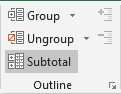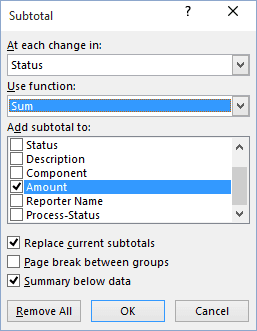# Creating Subtotals

Excel
Excel's ability to create automatic subtotals is a handy feature that can save you a great deal of time.

To use this feature, your list must be sorted, because the subtotals are inserted whenever the value in a specified field changes.

To insert subtotal formulas into a list automatically, move the cell pointer anywhere in the list and on the Data tab, in the Outline group, choose Subtotal:orThis dialog box offers the following choices:• At each change in - this drop-down list displays all fields in your list. The field that you choose must be sorted.
• Use function - this gives you a choice of 11 functions. You should normally use Sum (the default).
• Add subtotal to - this list box lists all the fields in your list. Place a checkmark next to the field or fields that you want to subtotal.
• Replace current subtotals - if this box is checked, any existing subtotal formulas are removed and replaced with the new subtotals.
• Page break between groups - if this box is checked, Excel inserts a manual page break after each subtotal.
• Summary below data - if this box is checked, the subtotals are placed below the data (the default). Otherwise, the subtotal formulas are placed above the totals.
• Remove All - this button removes all subtotal formulas in the list.

When you click OK, Excel analyzes the list and inserts formulas as specified - and creates an outline for you. The formulas all use the SUBTOTAL spreadsheet function.